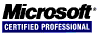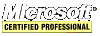# .NET C# Guide

## Variables

Examples of declaring several variables are here. There are six variables declared and four of them are initialized using the assignment operator (`=`).

``````int i = 123, x;
int j;
double d = 1.1;
char ch = 'A';
float f = 1f;
``````

Variables can also be initialized with other variables.

``````int i = 123;
int j = i + 10; // j contains 133
int x = i; // x contains 123
``````

## Operators

Operators are symbols that tell the compiler to perform a specific mathematical or logical manipulation. Basically, C# has four classes of operators: arithmetic, bitwise, relational and logical.

### Arithmetic Operators

operatoroperation
-subtraction
*multiplication
/division
%modulus
++increment
--decrement

The operators +, -, *, and / all work as expected. The % operator (modulus) returns the remainder of an integer division. Interestingly, it also works with floating-point types which is something that C/C++ cannot do.

#### Increment and Decrement

The operators -- and ++ decrement and increment by one, respectively. They come in two flavors: prefix and postfix. These operators only work on variables. The prefix one works as one would expect.

``````int num = 10;
int i;
i = ++num + 10; // i is 21, num is 11
i = --num + 10; // i is 20, num is 10``````

The postfix increment/decrement operators are not so obvious.

``````int num = 10;
int i;
i = num++ + 10; // i is 20, num is 11
i = num-- + 10; // i is 21, num is 10``````

### The Assignment Operator: =

Consider this example:

``````int a, b, c;
a = b = c = 10; // a, b, and c equal 10

int x, y, z = 3; // z equals 3
x = y = z; // x and y equal 3

z = z + 6; // z equals 9
``````

The statement `a = b = c = 10;` is an example of a chain of assignments.

#### Compound Assignments

Compound assignments simplify the coding of some assignment statments. Consider this example:

``````int i = 0;
i = i + 5; // i equals 5
i += 10; // i equals 15
``````

The arithmetic and logical assignment operators are: `+=` `+=` `*=` `/=` `&=` `%=` `^=` `|=`

### Relational Operators

operatoroperation
==equal to
!=not equal to
>greater than
<less than
>=greater than or equal
<=less than or equal

### Logical Operators

operatoroperation
&AND
|OR
^XOR
!NOT
||short-circuit OR
&&short-circuit AND

#### Short-circuit Operators

Short-circuit AND and short-circuit OR operators are special AND and OR operators. The only difference between the short-circuit and normal versions is that the short-circuit version will evaluate the second operand only when necessary while the normal version always evaluates the second operand.

### Bitwise Operators

operatoroperation
&AND
|OR
^XOR
<<shift left
>>shift right
~unary NOT

### The Conditional Operator: ?

The ? is a ternary operator because it requires three operands. It's syntax is: ```expression1 ? expression2 : expression3``` where expression1 is a bool and if true then the entire statement evaluates to expression2 otherwise it's expression3.

``````int x = 0, i = 10;
i = (x == 0) ? i : (i / x);
``````

### The Coalescing Operator: ??

The coalescing operator uses the syntax `??` to define a default value for the conversion in case the nullable type has a value of null. Here, `str2` receives a value of `string.Empty` because `str1` is null.

``````string str1 = null;
string str2 = str1 ?? string.Empty;
``````

The operators of C# are almost identical to the JavaScript ones with the exception of `>>>`, `===`, and `!==` as C# does not have these. And, values of `Infinity` and `NaN` do not exist in C#. Note that JavaScript only supports a few data types unlike C#. The C# operators are identical to the C/C++ ones.

#### Example Program Code

This example uses the short-circuit AND to prevent the numerator from being divided by the denominator which is set to zero.

``````using System;

namespace ConsoleApplication1
{
class Program
{
static void Main(string[] args)
{
int numerator = 100;
int denominator = 0; // CANNOT DIVIDE BY ZERO!!!

// first check that denominator is "not equal to" zero
// if it is not then divide numerator by denominator
if ((denominator != 0) && ((numerator / denominator) == 1))
Console.WriteLine("numerator and denominator are equal.");
else
Console.WriteLine("numerator and denominator are not equal.");
}
}
}
``````

This example uses the normal AND which will not prevent the numerator from being divided by the denominator which is set to zero. This will result in a divide-by-zero error which will crash the program.

``````using System;

namespace ConsoleApplication1
{
class Program
{
static void Main(string[] args)
{
int numerator = 100;
int denominator = 0; // CANNOT DIVIDE BY ZERO!!!

// first check that denominator is "not equal to" zero
// but also evaluate the second operand thereby dividing
// the numerator by the denominator crashing the program
if ((denominator != 0) & ((numerator / denominator) == 1))
Console.WriteLine("numerator and denominator are equal.");
else
Console.WriteLine("numerator and denominator are not equal.");
}
}
}
``````

### Operator Precedence

```Highest Precedence
() [] . ++(postfix) --(postfix) checked	new sizeof typeof unchecked
!	~	(cast)		+(unary)	-(unary) ++(prefix) --(prefix)
* / %
+ -
<< >>
< > <= >= is
== !=
&
^
|
&&
||
?:
= += -= *= /= %= &= |= ^=
Lowest Precedence
```

## Parentheses

Just like in algebra, parentheses increase the precendence of the operations contained with in them.

``````int i = 20 / 2 * 5; // i equals 50

int j = 20 / (2 * 5); // j equals 2
``````

## Type Casting

Casting instructs the compiler to convert one type into another. Put the cast inside parentheses as follows.

``````double d = 1000.1;

int i = (int)d; // (int) is the cast; now i equals 1000

byte b = (byte)i; // b cannot fit 1000 so data is lost (b equals 232 because 2 bits were lost)
``````

Also, casting can be done at runtime using the `as` keyword. If the cast succeeds then a reference to type is returned otherwise `null` is returned.

## Type Identification

It is possible to determine an object's type at runtime using the keywords `is` and `typeof`.

To determine if an object is a certain type use the `is` operator.

``````using System;

namespace ConsoleApplication1
{
class Program
{
static void Main(string[] args)
{
string s = string.Empty;
object o = s;
int[] n = { 1, 2, 3 };

if (s is string)
Console.WriteLine("s is a string");
else
Console.WriteLine("s is not a string");

if (s is object)
Console.WriteLine("s is an object");
else
Console.WriteLine("s is not an object");

if (o is string)
Console.WriteLine("o is a string");
else
Console.WriteLine("o is not a string");

if (o is object)
Console.WriteLine("o is an object");
else
Console.WriteLine("o is not an object");

if (n is string)
Console.WriteLine("n is a string");
else
Console.WriteLine("n is not a string");

if (n is object)
Console.WriteLine("n is an object");
else
Console.WriteLine("n is not an object");

if (n is Array)
Console.WriteLine("n is an Array");
else
Console.WriteLine("n is not an Array");

}
}
}
``````

Using `typeof`:

``````using System;

namespace ConsoleApplication1
{
class Program
{
static void Main(string[] args)
{
Type t;

t = typeof(Console);
Console.WriteLine(t.FullName);

t = typeof(Program);
Console.WriteLine(t.FullName);
}
}
}
``````
<< < [Page 3 of 7] > >>